Next: The LMS adaptive filter Up: number9 Previous: General principles

The Kalman filter

The simplest error signal that we can generate is just the difference between what we are getting out of the filter,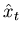, and what we expect to see,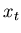,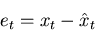(1)

For many problems an overshoot is just as bad as an undershoot, so we can use the mean square error as a cost function. There are lots of other cost functions that we could use, but this one is particularly convienient mathematically. The earliest adaptive filter derived from this error and cost function is the Wiener filter. Unfortunately, it is in FIR form and has coefficients that extend infinitely back in time. Except for certain periodic systems, this filter is not very practical. The filter can be rederived in IIR form, this is known as the Kalman filter. The discrete time, linear, form of this equation looks like,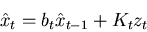(2)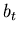, is a model of how the system goes from one time interval to the next. It is our best understanding of how the ideal system goes from a value at time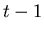to a value at time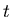.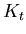is the Kalman gain, it is not controlled by the measurements directly, but instead is determined by how good you think the model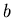is as compared to the quality of the observations.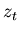is called the innovation, it is an estimate of what you think the error will be at time, given a measurement at time,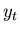, and a prediction of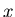at timebased upon the best estimate ofatextrapolated to timeby applying our model funtion,. The simplest example of how to calculate the innovation is,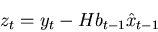(3)

where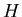is a function that may be necessary to convert the components ofto the components of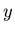(An example is the meteorological case of estimating the humidity (the) based upon wet-bulb and dry-bulb temperature measurements (the). Thefunction would have one component that converts humidity to wet-bulb temperature, and one for the conversion to dry-bulb). In an application, the innovation is a known function, like above, and the functionis known. What is not known is the gain,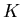; this must be calculated in parallel with the model estimation. The time varying gain is where the adaptative nature of the Kalman filter expresses itself.

In order to determine the equation that gives us the gain function, we have to spend some time with optimal estimation theory. I will not spend the time on this here, but just show the the result. In the scalar case, the gain function is: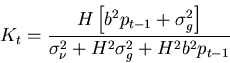(4)

Two of the new quantities,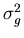and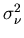, are the noise or error variances for the model,, and the measurements respectively. The first is a statement about how good you believe the model of the system is. The second quantifies how good you think your measurements are. Both of these quantities are presumed to be known. The third quantity,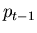, is the error covariance of the filter, it gives effectively the error bars of the current model output. This can be calculated given the gain,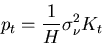(5)

To use the filter, each time a new observation () becomes available we calculate (3) and (4), and then use that information in (2) and (5).

The Kalman filter is frequently applied to systems whereandare multi-channel or vector systems. In this case the equations (2) through (5) are rewritten as matrix equations.Next: The LMS adaptive filter Up: number9 Previous: General principles
Skip Carter 2008-08-20